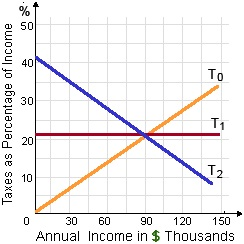quantity supplied

how do you calculate quantity supplied

#### Related Questions in Macroeconomics

• ##### Q :Sources of demand for foreign currency

State main sources of demand for foreign currency?

Answer: The four main sources of demand for foreign currency are as follows:

A) To buy services and goods from other countries.

• ##### Q :Zero primary deficits What points out

What points out zero primary deficits?

Answer: Zero primary deficits signify that the government has to resort to borrowings simply to make interest payments.

• ##### Q :Limitation of credit availability What

What occurs to economy, when credit availability is limited and credit is made costlier?

Answer: Aggregate demands falls

• ##### Q :Formula for Fiscal deficit Fiscal

Fiscal deficit: Fiscal deficit is stated as the surplus of total expenditure over total receipts, apart from borrowings.

Fiscal deficit = Total expenditure (Rev. Exp. + Cap. Exp.) – Total Receipts

• ##### Q :What are various economic growth

Economic growth is generally defined as a sustained increase in per capital national output over a long period of time. It implies that for economic growth of a nation, the rate of increase in its total output must be greater than the rate of population growth. It ma

• ##### Q :Market price decrement according to

When heroin were legalized, in that case the: (w) market price of heroin would drop considerably. (x) demand would raise although supply would decrease. (y) demand would decrease but supply would increase. (z) price of cocaine would raise.

##### Q :Creation of assets or reduction of

Illustrate which budget expenses does not result in the creation of assets or reduction of liability. Give illustrations too.

• ##### Q :Transactions demand for money The basic

The basic determinant of the transactions demand for money is the

• ##### Q :Fiscal Monetary changes With the

With the general equilibrium framework in place, the stage is now set for introducing fiscal and monetary changes and analysing their effects on the general equilibrium. We will first introduce a fiscal change in the form of increase in deficit-financed expenditure, a

• ##### Q :Problem on tax system In the figure

In the figure shown below, line T0 depicts a tax system which is: (1) Progressive. (2) Regressive. (3) Proportional. (4) Unbiased. (5) Recessive.18,76,764

Questions